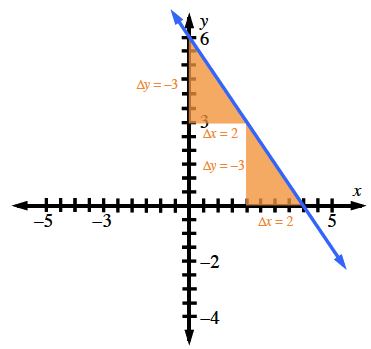### Home > CCG > Chapter 6 > Lesson 6.1.5 > Problem6-49

6-49.

On graph paper, graph the line $y=-\frac{3}{2}x+6$. Name the $x\text{-}$ and $y\text{-intercepts}$.

A good place to start: $(0,6)$.

$\text{slope}=\frac{\Delta y}{\Delta x}=-\frac{3}{2}$

Look at the graph. Where does the line cross the $x\text{-axis}$, and where does it cross the $y\text{-axis}$?